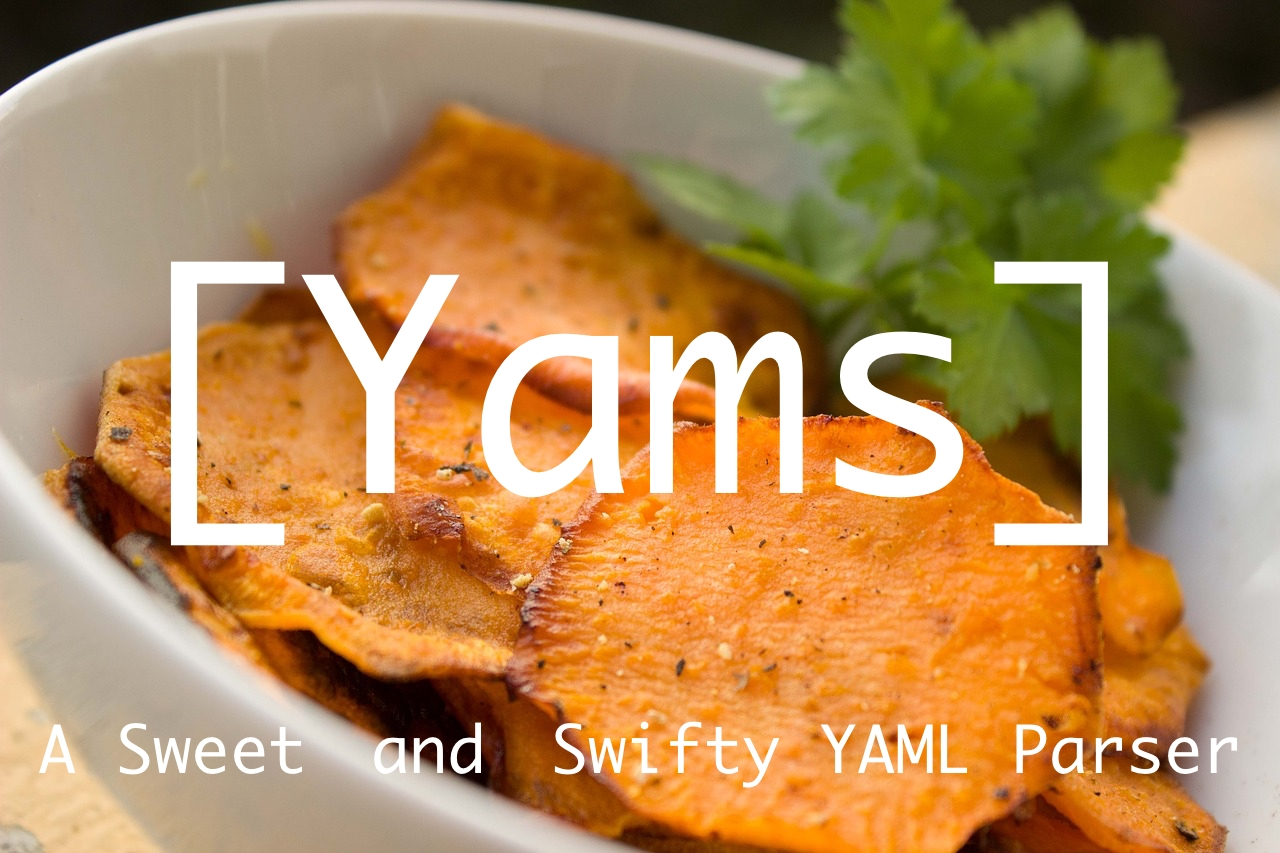## Yams

### master

A Sweet and Swifty YAML parser.

# YamsA sweet and swifty YAML parser built on LibYAML.## Installation

Building Yams requires Xcode 10.x or a Swift 4.1+/5.x toolchain with the Swift Package Manager.

### Swift Package Manager

Add `.package(url: "https://github.com/jpsim/Yams.git", from: "2.0.0")` to your `Package.swift` file's `dependencies`.

### CocoaPods

Add `pod 'Yams'` to your `Podfile`.

### Carthage

Add `github "jpsim/Yams"` to your `Cartfile`.

## Usage

Yams has three groups of conversion APIs: one for use with `Codable` types, another for Swift Standard Library types, and a third one for a Yams-native representation.

#### `Codable` types

• Codable is an encoding & decoding strategy introduced in Swift 4 enabling easy conversion between YAML and other Encoders like JSONEncoder and PropertyListEncoder.
• Lowest computational overhead, equivalent to `Yams.Node`.
• Encoding: `YAMLEncoder.encode(_:)` Produces a YAML `String` from an instance of type conforming to `Encodable`.
• Decoding: `YAMLDecoder.decode(_:from:)` Decodes an instance of type conforming to `Decodable` from YAML `String`.
```import Foundation
import Yams

struct S: Codable {
var p: String
}

let s = S(p: "test")
let encoder = YAMLEncoder()
let encodedYAML = try encoder.encode(s)
encodedYAML == """
p: test

"""
let decoder = YAMLDecoder()
let decoded = try decoder.decode(S.self, from: encodedYAML)
s.p == decoded.p```

#### Swift Standard Library types

• The type of Swift Standard Library is inferred from the contents of the internal `Yams.Node` representation by matching regular expressions.
• This method has the largest computational overhead When decoding YAML, because the type inference of all objects is done up-front.
• It may be easier to use in such a way as to handle objects created from `JSONSerialization` or if the input is already standard library types (`Any`, `Dictionary`, `Array`, etc.).
• Encoding: `Yams.dump(object:)` Produces a YAML `String` from an instance of Swift Standard Library types.
• Decoding: `Yams.load(yaml:)` Produces an instance of Swift Standard Library types as `Any` from YAML `String`.
```// [String: Any]
let dictionary: [String: Any] = ["key": "value"]
let mapYAML: String = try Yams.dump(object: dictionary)
mapYAML == """
key: value

"""
let loadedDictionary = try Yams.load(yaml: mapYAML) as? [String: Any]

// [Any]
let array: [Int] = [1, 2, 3]
let sequenceYAML: String = try Yams.dump(object: array)
sequenceYAML == """
- 1
- 2
- 3

"""
let loadedArray: [Int]? = try Yams.load(yaml: sequenceYAML) as? [Int]

// Any
let string = "string"
let scalarYAML: String = try Yams.dump(object: string)
scalarYAML == """
string

"""
let loadedString: String? = try Yams.load(yaml: scalarYAML) as? String```

#### `Yams.Node`

• Yams' native model representing Nodes of YAML which provides all functions such as detection and customization of the YAML format.
• Depending on how it is used, computational overhead can be minimized.
• Encoding: `Yams.serialize(node:)` Produces a YAML `String` from an instance of `Node`.
• Decoding `Yams.compose(yaml:)` Produces an instance of `Node` from YAML `String`.
```var map: Yams.Node = [
"array": [
1, 2, 3
]
]
map.mapping?.style = .flow
map["array"]?.sequence?.style = .flow
let yaml = try Yams.serialize(node: map)
yaml == """
{array: [1, 2, 3]}

"""
let node = try Yams.compose(yaml: yaml)
map == node```